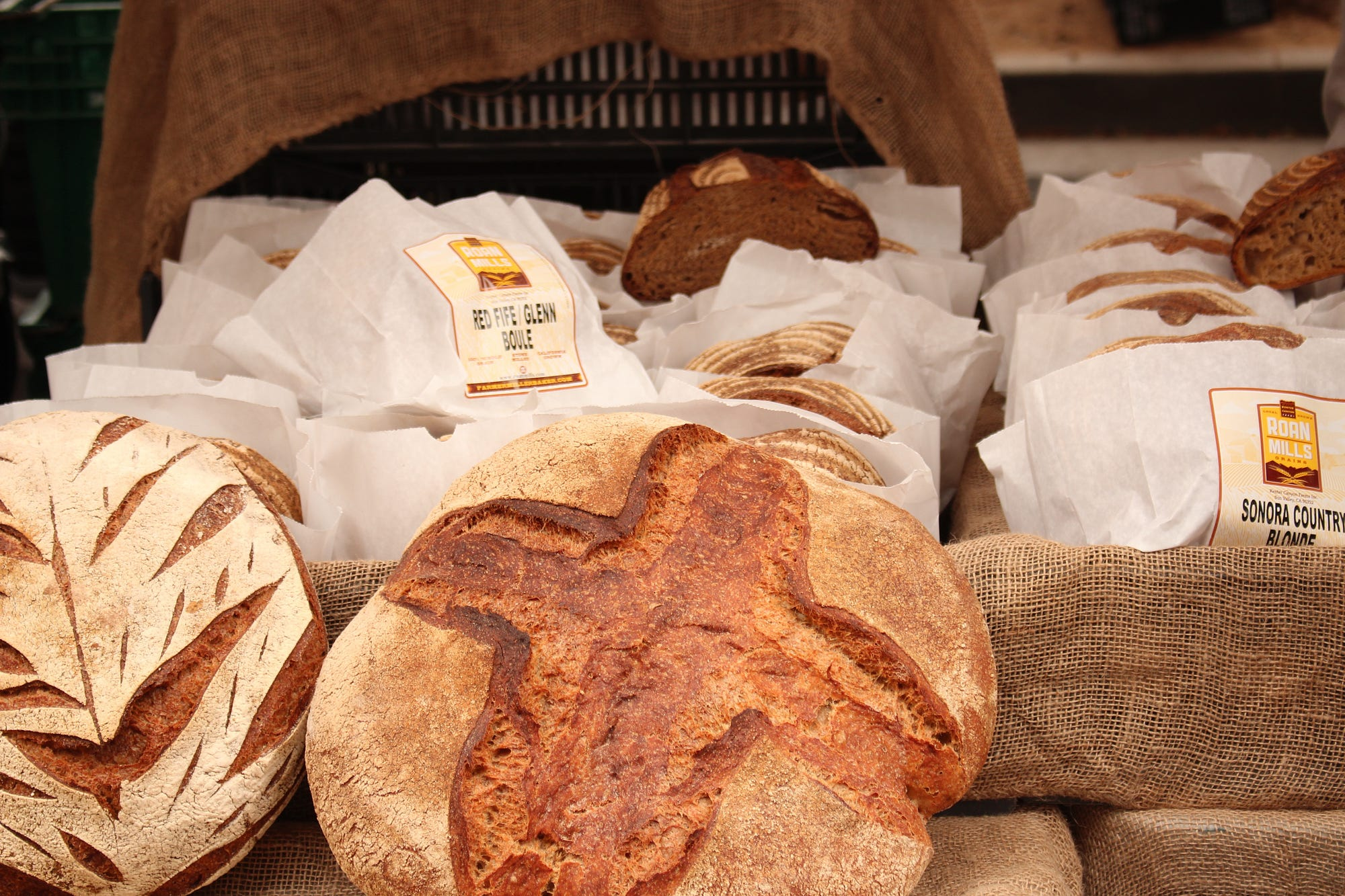# MBA for Breakfast by George Wong

Posted on# MBA For Breakfast or also known as the Market Basket Analysis

Written by George WongSo recently, I was fortunate enough to work on a project that involves doing market basket analysis but obviously I wouldn’t be able to discuss my work on Medium. So instead, I try to look for suitable datasets on Kaggle.com. Which I managed to find one here AND applied whatever that I know to make it happen! Also Shout-out to Susan Li for her wonderful work on MBA, which can be found here!

So what is a Market Basket Analysis? According to the book Database Marketing:

Market basket analysis scrutinizes the products customers tend to buy together, and uses the information to decide which products should be cross-sold or promoted together. The term arises from the shopping carts supermarket shoppers fill up during a shopping trip.

On a side note, Market Basket is also a chain of 79 supermarkets in New Hampshire, Massachusetts, and Maine in the United States, with headquarters in Tewksbury, Massachusetts (Wikipedia).

Normally MBA is done on transaction data from the point of sales system on a customer level. We can use MBA to extract interesting association between products from the data. Hence its output consists of a series of product association rules: for example, if customers buy product A they also tend to buy product B. We will follow the three most popular criteria evaluating the quality or the strength of an association rule (will get back to this later).

Getting the right packages (Python):

```import pandas as pd
import numpy as np
import seaborn as sns
import matplotlib.pyplot as plt
from mlxtend.frequent_patterns import apriori
from mlxtend.frequent_patterns import association_rules
import mlxtend as ml```

```bread = pd.read_csv(r"D:\Downloads\BreadBasket_DMS.csv")

“Data set containing 15’010 observations and more than 6.000 transactions from a bakery.” More information on the variables can be found in Kaggle.

```sns.countplot(x = 'Item', data = bread, order = bread['Item'].value_counts().iloc[:10].index)
plt.xticks(rotation=90)```

It seems like coffee are the hottest item in the dataset, I guess everybody wants a cup of hot coffee in the morning perhaps.

#### How many items are sold daily?Time to get right into the MBA itself! Kudos to Chris Moffitt on the awesome guide and tutorial of MBA using python.

We will be using MLxtend library’s Apriori Algorithm for extracting frequent item sets for further analysis. The `apriori`function expects data in a one-hot encoded pandas DataFrame. Thus your dataframe should look like this:

First, We’ll group the `bread` dataframe accordingly and display the count of items then we need to consolidate the items into 1 transaction per row with each product 1 hot encoded. Which would result in the table above!

`df = bread.groupby(['Transaction','Item']).size().reset_index(name='count')`
```basket = (df.groupby(['Transaction', 'Item'])['count']
.sum().unstack().reset_index().fillna(0)
.set_index('Transaction'))```
```#The encoding function
def encode_units(x):
if x <= 0:
return 0
if x >= 1:
return 1```
`basket_sets = basket.applymap(encode_units)`

After that, we will generate frequent item sets with a minimum support of at least 1% as this a more favourable support level that could show us more results.

```frequent_itemsets = apriori(basket_sets, min_support=0.01, use_colnames=True)
rules = association_rules(frequent_itemsets, metric="lift")
rules.sort_values('confidence', ascending = False, inplace = True)

#### The end

Remember I told y’all that we’ll get back to the three most popular criteria evaluating the quality or the strength of an association rule. There are support, confidence and lift:
1. Support is the percentage of transactions containing a particular combination of items relative to the total number of transactions in the database. The support for the combination A and B would be,

P(AB) or P(A) for Individual A

2. Confidence measures how much the consequent (item) is dependent on the
antecedent (item). In other words, confidence is the conditional probability of the consequent given the antecedent,

P(B|A)

where P(B|A) = P(AB)/P(A)

3. Lift (also called improvement or impact) is a measure to overcome the
problems with support and confidence. Lift is said to measure the difference — measured in ratio — between the confidence of a rule and the expected confidence. Consider an association rule “if A then B.” The lift for the rule is defined as

P(B|A)/P(B) or P(AB)/[P(A)P(B)].

As shown in the formula, lift is symmetric in that the lift for “if A then B” is the same as the lift for “if B then A.”
4. Each criterion has its advantages and disadvantages but in general we would like association rules that have high confidence, high support, and high lift.

As a summary,

Confidence = P(B|A)

Support = P(AB)

Lift = P(B|A)/P(B)

From the output, the lift of an association rule “if Toast then Coffee” is 1.48 because the confidence is 70%. This means that consumers who purchase Toast are 1.48 times more likely to purchase Coffee than randomly chosen customers. Larger lift means more interesting rules. Association rules with high support are potentially interesting rules. Similarly, rules with high confidence would be interesting rules as well.

Reference: Can be found throughout the article 🙂

Source code in Jupyter Notebook here!

Link to the original article can be found here